# Power | Need, derivation, Mechanical Advantage class -11

• 151
•
•
•
•
•
•
•
151
Shares

Do we know that how work is defined for a force whether it’s a constant force or variable force, here we will discuss what is power? What’s need to define Power in Physics? What’s the expression of power for a given force and Power for net force acting on a system. Relationship of Mechanical Advantage also Powers of a pump/engine. So let’s start…

# Power

## Need to define Power

We know that work done by a force depends on the amount of force and displacement caused by the force. Now suppose a situation that two persons lift a block of the same mass to the same height, which means they do work against gravity, and since the force and Displacement are the same the work done by both is the same.

Now, think what if one of them is lifting two times the block to height “h” in a second, and another person lifting the same block to the same height, only one time in a second. Also, if we consider lifting blocks once both are lifting with the same force and displacement of block is the same and work done is the same.

In the above observation, we say that the person lifting twice a second is consuming his energy faster than others who lift once a second. So we can define a term related to work and time, and we say qualitatively that the rate of energy consumption or the rate of doing work is Power. Now, we will see how Power is calculated.

## Defining Power

In physics, power is defined as the rate of doing work, or since energy is being consumed while doing work we can say that power is the rate of consumption of energy. The rate of any quantity means its ratio with time hence rate of doing work is the ratio of work with the time and its power.

### Power for a given force

#### Power of Translational work

When a system is being experienced by a given force and the system is doing translational motion then the work defined is the translational work and the rate of doing translational work is Translational power we can say.

Let’s say that a force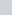is acting on a body.
From definition of power

(1)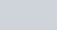(2)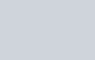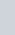is velocity

(3)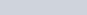#### Power of Rotational work

For the case of torque due to a given force.

If system is undergoing rotational motion by action of a force then the work defined is rotational work and rate of doing of rotational work is rotational power.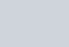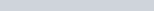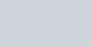### Power of net force

#### Derivation of expression for power of net force

From Work-Energy theorem we know that work done on a system by resultant force means net force equals to the change in kinetic energy of system.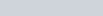(4)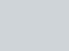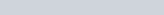(5)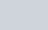here v is speed it’s not velocity , and the speed is changed by only the tangential component of acceleration which is tangential to velocity and the perpendicular component of acceleration changes direction of object not speed .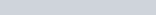(6)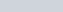Similarly, we can derive rotational forces also.

## Average and Instantaneous Power

Suppose a person lifts a bag from the ground floor to the top floor of a high building, here the person is doing work against gravity and it takes considerable time for him to take the bag to the topmost floor in this duration sometimes the person lifts the bag with larger forces initially then he may be tired and later on Maybe lifting with comparatively lesser forces.

What we are concluding is That for a time duration at different times the power can be different but when talking for power in a time duration we define average power

(7)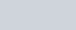It means on an average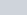amount of power is driven.

Now, think that if the time interval is very small so that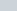change in time is almost zero we can say that we are talking for a particular instant of time, in this case, the average power becomes instantaneous power if the time interval is very minute.

Mathematically,

Instantaneous Power is the limiting value of average power when time intervalapproaches zero.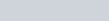(8)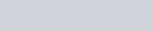## Power of Pump/Engine

Consider a tank that stores water located at a height “h” from the motor, we define a new term which is for the rate of pumping of the mass of water from the motor as Mass Flow Rate which equals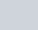“dm” represents a very small mass.Now let’s find the power of a motor. Now think for a “dm” mass of water going upwards and it’s being acted by two forces – force by gravity and force by motor new we are applying work-energy-theorem for the duration the dm mass of water is pumped from motor to tank.

(9)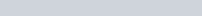Assume that water is driven at a constant speed . And for this condition the initial and final speed for this mass is same hence the change in kinetic energy is zero . Hence equation (9) becomes :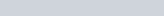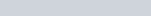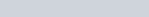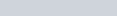above expression is work done by motor and its rate of doing work will be power of motor.

(10)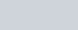Suppose A system and If in this system there is no loss of energy then the total power input to the system is equal to the total power output. This principle gives us the useful relationship of Mechanical advantage.Let there’s a point “a” on a system at which power is input to the system and a point “b” on the system through which power is being output from the system.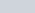are the velocities of point a and point b respectively and if there’s no loss in energy then the power input will always equal to the power output.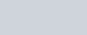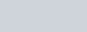The above expression expresses the mechanical advantage of the system as power stated is for translation motion hence the mechanical advantage is also For this type of motion system.

Similarly the relationship for the systems in rotational motion.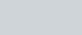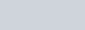These relations are very useful and applicable. Stay tuned with Laws Of Nature for more useful and interesting content.